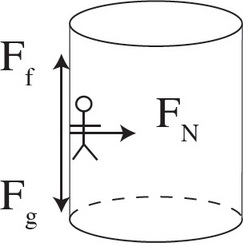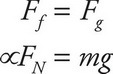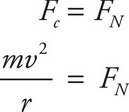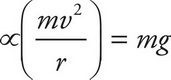# AP Physics 1 Question 361: Answer and Explanation

### Test Information

Question: 361

9. The Gravitron is a carnival ride that looks like a large cylinder. People stand inside the cylinder against the wall as it begins to spin. Eventually, it is rotating fast enough that the floor can be removed without anyone falling. Given that the coefficient of friction between a person's clothing and the wall is μ, the tangential speed is v, and the radius of the ride is r, what is greatest mass that a person can be to safely go on this ride?

• A. μv2/(rg)
• B. r2v2/(µg)
• C. rg/(µv2)
• D. None of the above

Explanation:

D

First, draw a free-body diagram:In order to stay in place, the force of friction needs to have a magnitude equal to the force of gravity's.Next, solve for the normal force. This is possible because the person is undergoing uniform circular motion, so set the normal force equal to centripetal force.Finally, substitute this value into the previous result.Notice that the mass actually drops out of the equation. The only variables that matter are the coefficient of friction, speed, and the radius.